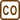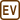## Luminosity of the light bulb

Let’s consider a light bulb with the parameters 60 W and 230 V. Determine the ratio of the radiant fluxes for this specific light bulb in case when its connected on 230 V and in the case when the voltage drops to 210 V. Discuss the corresponding ratio of luminous fluxes.

Use the results from the task Radiation and current – voltage characteristic of a light bulb.

• #### Hint

From the parameters listed on the bulb calculate the current that flows through the bulb with given voltage.

Use obtained values to determine the constant C in the relation between the current and the voltage on the bulb in the result of the task Radiation and current – voltage characteristic of a light bulb

• #### Analysis

We will determine the amount of current flow during the given power and voltage from the light bulb’s parameters. This will allow us to find the constant for the relation between the current and the voltage which we derived from the task Radiation and current – voltage characteristic of a light bulb. With the constant we can later on determine the current and the power on the bulb with lower voltage. In the steady state the electric power and luminosity are equal therefore the ratio of luminosities is equal to the ratio of electric powers.

• #### Solution

From the given power P and the voltage U on the bulb we can determine the current that flows through the bulb

$P=UI\,\Rightarrow\,I=\frac{P}{U}=\frac{60}{230}\,\mathrm{A}=0.26\,\mathrm{A} .$

We will use the results from the task Vyzařování a voltampérová charakteristika žárovky and determine the constant C for this bulb to the relation between the current and the voltage

$I=CU^{\frac{3}{5}}\,\Rightarrow\,C=\frac{I}{U^{\frac{3}{5}}}=\frac{\frac{P}{U}}{U^{\frac{3}{5}}}=\frac{P}{U^{\frac{8}{5}}} .$

We can determine the electric current I1 that flows through the bulb with lower voltage U1 using the constant C

$I_1=CU_{1}\,^{\frac{3}{5}}$

and the power of the light bulb P1 with lower voltage

$P_1=U_1I_1=U_1CU_{1}\,^{\frac{3}{5}}=CU_{1}\,^{\frac{8}{5}}=\frac{P}{U^{\frac{8}{5}}}U_{1}\,^{\frac{8}{5}}=P\left(\frac{U_1}{U}\right)^{\frac{8}{5}} .$

Since in steady state the luminosity is equal to electric power we can write

$\frac{P_{\mathrm{radiation1}}}{P_{\mathrm{radiation}}}=\frac{P_1}{P}=\left(\frac{U_1}{U}\right)^{\frac{8}{5}}=\left(\frac{210}{230}\right)^{\frac{8}{5}}=0.86=86\% .$
• #### Solution – ratio of the luminous fluxes

While decreasing the voltage on the light bulb the electric current and the electric power decrease too. This means that also the temperature of the light bulb’s filament will decrease. When the filament’s temperature is decreased the total radiated power is decreased but due to this phenomena the radiation spectrum will move more to the infrared region. This means that with the lower temperature the infrared (thermal) region dominates over light. This is the reason why the ratio of the luminous fluxes for both voltages will be even smaller than the ratio of the luminosities.